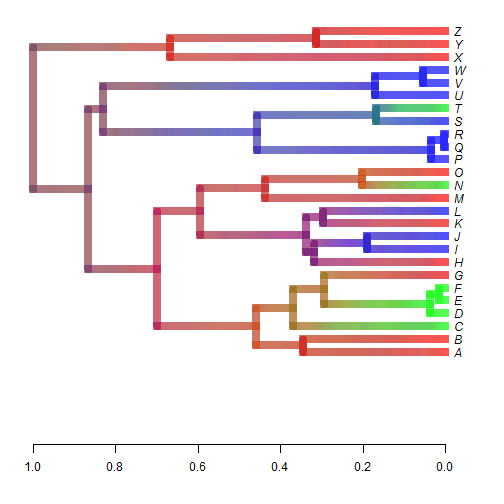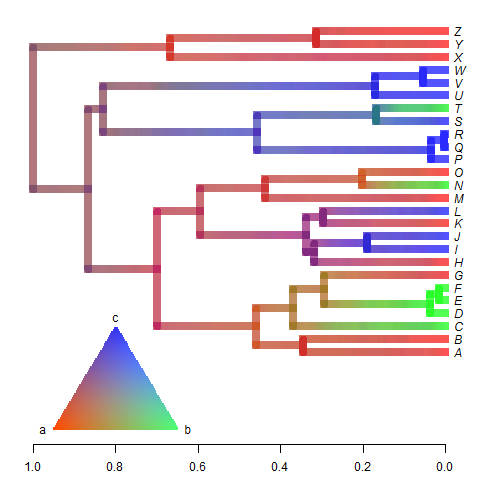## Thursday, September 21, 2017

### `densityMap`-like plot for a 3-state discrete character

Today I received the following email:

“I'm a postdoc working on a project to reconstruct the ancestral … with … at the University of …. We've been using phytools and getting nice results but we've run into one issue we were hoping you might be able to help us with. We're interesting in generating a posterior density map for our data, but, as far as we can tell, the function `densityMap` can only be used for binary characters. Do you know of a way to do a densityplot with non-binary characters (3 character states) or can you recommend a workaround? Any assistance you can provide would be greatly appreciated. Thank you for your time and your help.”

This sounds like something I would love to implement, so perhaps I will; however in the meantime there is a kind of workaround that can be done using `densityTree` (not `densityMap`, in fact) in the phytools package as follows:

``````library(phytools)
tree
``````
``````##
## Phylogenetic tree with 26 tips and 25 internal nodes.
##
## Tip labels:
##  A, B, C, D, E, F, ...
##
## Rooted; includes branch lengths.
``````
``````x
``````
``````## A B C D E F G H I J K L M N O P Q R S T U V W X Y Z
## a a b b b b a a c c a c a b a c c c c b c c c a a a
## Levels: a b c
``````
``````trees<-make.simmap(tree,x,model="ER",nsim=100)
``````
``````## make.simmap is sampling character histories conditioned on the transition matrix
##
## Q =
##            a          b          c
## a -1.7161484  0.8580742  0.8580742
## b  0.8580742 -1.7161484  0.8580742
## c  0.8580742  0.8580742 -1.7161484
## (estimated using likelihood);
## and (mean) root node prior probabilities
## pi =
##         a         b         c
## 0.3333333 0.3333333 0.3333333
``````
``````## Done.
``````
``````trees
``````
``````## 100 phylogenetic trees with mapped discrete characters
``````
``````colors<-setNames(c(rgb(1,0,0),rgb(0,1,0),rgb(0,0,1)),
letters[1:3])
densityTree(trees,method="plotSimmap",lwd=8,nodes="intermediate",
colors=colors,ylim=c(-5,26),compute.consensus=FALSE)
``````OK, so now we're part of the way there - but what about our legend?

Well, the following is a 'hack' - and would have to be adapted in its coordinates & so on for any particular plot - but hopefully you can see the idea:

``````densityTree(trees,method="plotSimmap",lwd=8,nodes="intermediate",
colors=colors,ylim=c(-5,26),compute.consensus=FALSE)
## make a triangle of cell coordinates
cells.x<-cells.y<-vector()
k<-1
asp<-abs(diff(par()\$usr[4:3])/diff(par()\$usr[2:1]))*par()\$pin/par()\$pin
for(i in 1:50){
for(j in i:(100-i+1)){
cells.x[k]<-0.65+(j-1)*0.3/100
cells.y[k]<-(-5)+(i-1)*2*0.3*asp/100
k<-k+1
}
}
## draw points
dx<-diff(range(cells.x))
dy<-diff(range(cells.y))
red.x<-max(cells.x)
red.y<-min(cells.y)
red<-1-sqrt((((cells.x-red.x)/dx)^2+((cells.y-red.y)/dy)^2)/2)
green.x<-min(cells.x)
green.y<-min(cells.y)
green<-1-sqrt((((cells.x-green.x)/dx)^2+((cells.y-green.y)/dy)^2)/2)
blue.x<-min(cells.x)
blue.y<-max(cells.y)
blue<-1-sqrt((((cells.x-blue.x)/dx)^2+((cells.y-blue.y)/dy)^2)/2)
points(cells.x,cells.y,col=rgb(red/max(red),green/max(green),blue/max(blue)),
pch=15,cex=0.5)
text(0.95,-5,"a",pos=2)
text(0.65,-5,"b",pos=4)
text((0.95-0.65)/2+0.65,max(cells.y),"c",pos=3)
``````OK, it's not perfect - but perhaps something to work from!

More later.

The tree & data were simulated as follows:

``````tree<-pbtree(n=26,tip.label=LETTERS,scale=1)
Q<-matrix(c(-1,0.5,0.5,0.5,-1,0.5,0.5,0.5,-1),
3,3,dimnames=list(letters[1:3],letters[1:3]))
x<-as.factor(sim.history(tree,Q)\$states)
``````